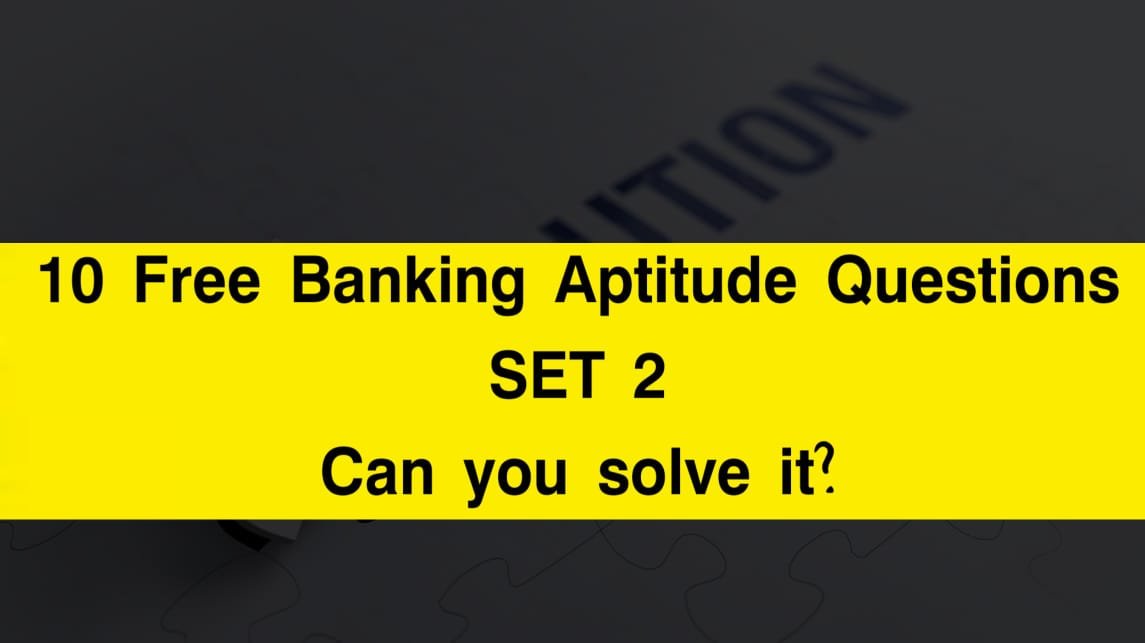# 10 Banking Aptitude Questions (Number Series) – SET 2 – Can you solve them? Try it!

Aptitude Questions are common in various competitive exams, including Banking exams. It is an important part of almost all banking exams like IBPS PO, IBPS Clerk, SBI PO, and many more.

In this article, we have brought to you another 10 Free Banking aptitude Questions with detailed answers and explanations so that you can solve these and prepare for various online exam preparation, various interviews, Logical Reasoning Categories online tests, and much more.

Here is the set:

## 10 NUMBER SERIES BANKING APTITUDE QUESTIONS

### (Q1-Q5) What will come in place of the blank (__) in each of the following series?

#### Q1. 9, 5, 6, 10.5, 23, __

OPTIONS

A. 50

B. 65

C. 60

D. 35

E. 75

ANS: C. 60

SOLUTION:

9 * 0.5 + 0.5 = 5
5 * 1 + 1 = 6
6 * 1.5 + 1.5 = 10.5
10.5 * 2 + 2 = 23
23 * 2.5 + 2.5 = 60
Therefore with this logic, the answer is 60.

#### Q2.17, 98, 26, __ , 35, 80

OPTIONS

A. 79

B. 75

C. 59

D. 89

E. 49

ANS: D. 89

SOLUTION:

26 -17 = 9
35 – 26 = 9
98 – 9 = 89
Therefore with this logic, the answer is 89.

#### Q3. 47, 78, 115, 164, 231, __

OPTIONS

A. 270

B. 299

C. 322

D. 351

E. 375

ANS: C. 322

SOLUTION:

47 47 + 31 = 78
78 + (31 + 6) = 115
115 + (37 + 12) = 164
164 + (49 + 18) = 231
231 + (67 + 24) = 322
Therefore with this logic, the answer is 322.

#### Q4. 7, 4.5 ,5.5, 12, 49, __

OPTIONS

A. 93

B. 149

C. 79

D. 245

E. 393

ANS: E. 393

SOLUTION:

7 × 0.5 + 1 = 4.5
4.5 × 1 + 1 = 5.5
5.5 × 2 + 1 = 12
12 × 4 + 1 = 49
49 × 8 + 1 = 393
Therefore the ANS is 393

#### Q5.68, 117, 61, 124, 54, __

OPTIONS

A. 131

B. 151

C. 141

D. 161

E. 101

ANS: A. 131

SOLUTION:

68–7=61
61–7=54
117+7=124
124+7=131
Therefore the ANS is 131

### (Q6-Q10) A number in the series is wrong. Find the wrong number.

#### Q6. 17, 23, 35, 55, 85, 125

OPTIONS

A. 17

B. 35

C. 55

D. 85

E. 125

ANS: E. 125

SOLUTION:

17+2×3=23
23+3×4=35
35+4×5=55
55+5×6=85
85+6×7=127
Hence the wrong no. is 125

#### Q7. 7, 37, 91, 167, 271, 397

OPTIONS

A. 7

B. 37

C. 167

D. 271

E. 91

ANS: C. 167

SOLUTION:

7=2^3–1^3 37=4^3–3^3
91=6^3–5^3
167≠8^3–7^3=169
271=10^3–9^3
397=12^3–11^3
So the wrong no. is 167

#### Q8. 1, 8, 22, 45, 71, 106, 148

A. 8

B. 22

C. 45

D. 71

E. 106

ANS: C. 45

SOLUTION:

1+7=8
8+14=22
22+21=43≠45
43+28=71
71+35=106
106+42=148
So the wrong no. is 45

#### Q9. 36,  20,  12,  8,  6,  5.5,  4.5

OPTIONS

A. 36

B. 20

C. 12

D. 6

E. 5.5

ANS: E. 5.5

SOLUTION:

36
20–8=12
12–4=8
8–2=6
6 – 1 = 5 which is not = 5.5
5–0.5=4.5
Hence wrong no. is 5.5

#### Q10. 28, 66, 128, 224, 348, 518

OPTIONS

A. 28

B. 66

C. 128

D. 224

E. 348

ANS: D. 224

SOLUTION:

3^3+1=28
4^3+2=66
5^3+3=128
6^3+4=220
7^3+5=348
8^3+6=518
Hence the wrong no.is 224

How many of the above questions have you solved?

Comment them down below. Also, comment down the time taken to solve them and beat it in the next SET.